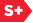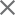### Proceedings Paper

The n-dimensional polyharmonic local sine transform on rectangular domains
Format Member Price Non-Member Price
PDF \$17.00 \$21.00

Paper Abstract

The polyharmonic local sine transform is a recently introduced transform that can be used to compress and interpolate datasets. This transform is based on both Fourier theory and the solution to the polyharmonic equation (e.g. Laplace equation, biharmonic equation). The efficiency of this transform can be traced to theorems from Fourier analysis that relate the smoothness of the input function to the decay of the transform coefficients. This decay property is crucial and allows us to neglect high order coefficients. We will discuss the case of the Laplace sine transform. We introduce an arbitrary dimensional version of this transform for datasets sampled on rectangular domains and generalize the 2-dimensional algorithm to n dimensions. Since the efficiency of the Laplace sine transform relies on the smoothness of the data, we cannot directly apply it to discontinuous data. In order to deal with this, we have developed a segmentation algorithm that allows us to isolate smooth regions from those with discontinuities. The result is a transform that will locally adapt to the features in a dataset,allowing us to perform meaningful tests on real, discontinuous datasets.

Paper Details

Date Published: 17 September 2005
PDF: 12 pages
Proc. SPIE 5914, Wavelets XI, 591425 (17 September 2005); doi: 10.1117/12.618626
Show Author Affiliations
Noel T. Smith, Univ. of California, Davis (United States)
Naoki Saito, Univ. of California, Davis (United States)

Published in SPIE Proceedings Vol. 5914:
Wavelets XI
Manos Papadakis; Andrew F. Laine; Michael A. Unser, Editor(s)